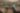# Convergence of Sparse Variational Inference in Gaussian Processes Regression

Date July 22, 2020
Authors David R. Burt, Carl Edward Rasmussen, Mark van der Wilk

Gaussian processes are distributions over functions that are versatile and mathematically convenient priors in Bayesian modelling. However, their use is often impeded for data with large numbers of observations, N, due to the cubic (in N) cost of matrix operations used in exact inference. Many solutions have been proposed that rely on M ≪ N inducing variables to form an approximation at a cost of O(NM^2). While the computational cost appears linear in N, the true complexity depends on how M must scale with N to ensure a certain quality of the approximation. In this work, we investigate upper and lower bounds on how M needs to grow with N to ensure high quality approximations. We show that we can make the KL-divergence between the approximate model and the exact posterior arbitrarily small for a Gaussian-noise regression model with M ≪ N. Specifically, for the popular squared exponential kernel and D-dimensional Gaussian distributed covariates, M = O((logN)^D) suffice and a method with an overall computational cost of O􏰁(N(log N)^2D(log log N)^2)􏰂 can be used to perform inference.

View the paper

Share
,,